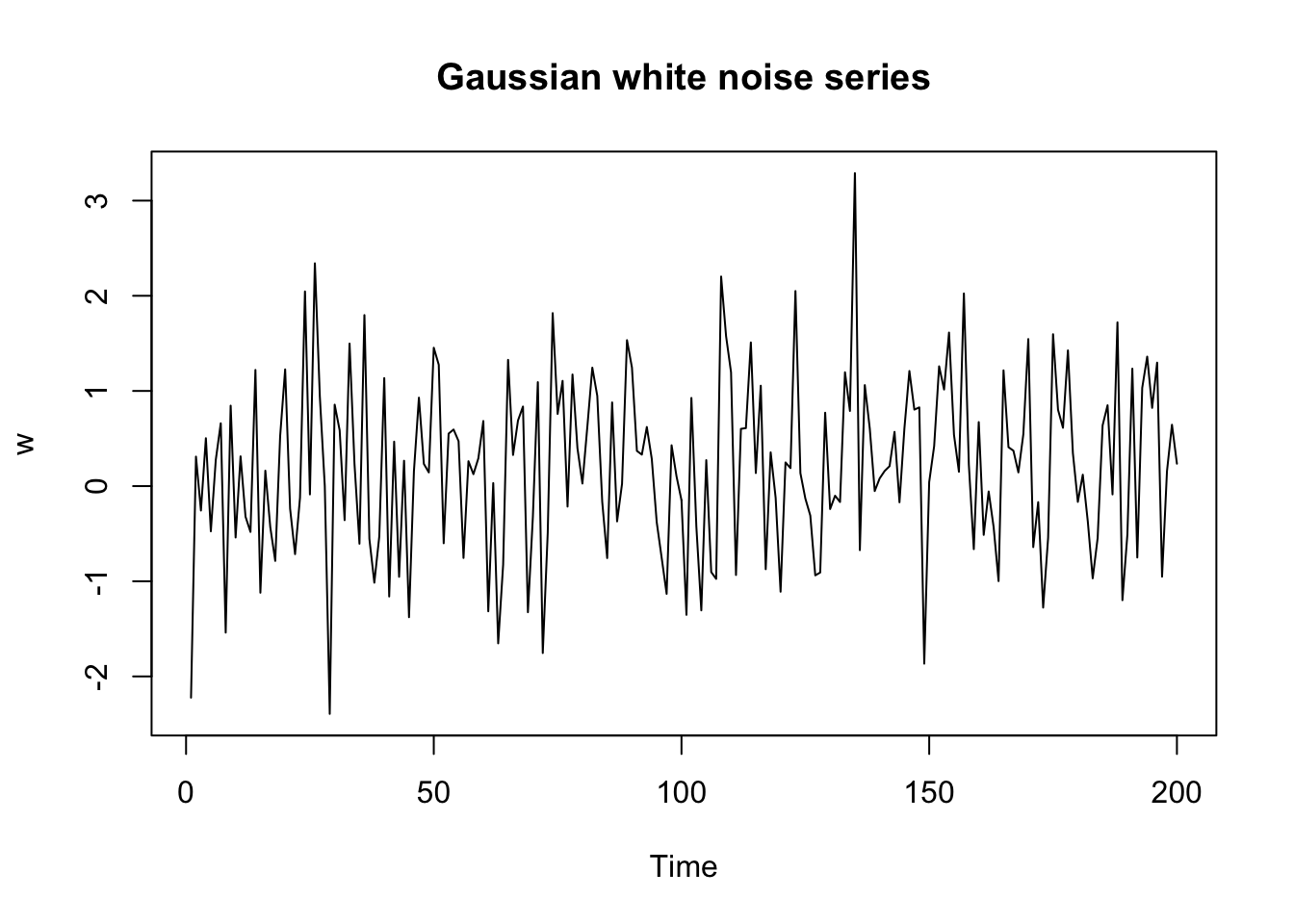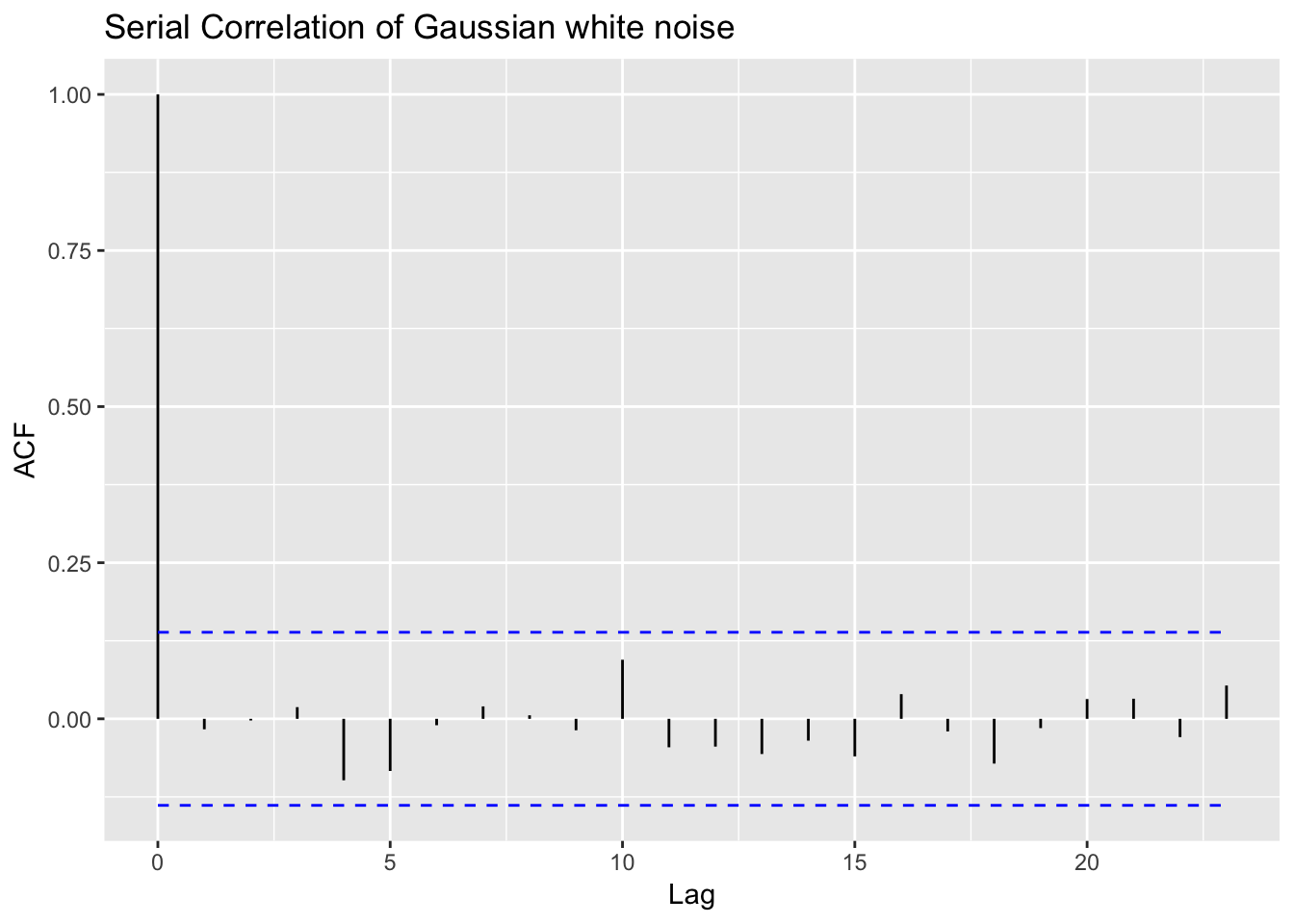If the random variables which make up $$y_t$$ are uncorrelated, have means 0 and variance $$\sigma^2$$, then $$y_t$$ is stationary with the autocovariance function of

$\gamma_w(s,t) = \text{cov}(w_s, w_t)= \begin{cases} \sigma^2_w & s=t \\ 0 & \text{otherwise} \end{cases}$

This type of series is referred to as white noise. The designation white originates from the analogy with white light and indicates that all possible periodic oscillations are present with equal strength (Shumway and Stoffer 2011).

A particularly useful white noise series is Gaussian white noise, wherein the $$w_t$$ are independent identically distributed (iid) normal random variables, with mean 0 and variance $$\sigma^2$$

$w_t \sim \text{iid } N(0, \sigma^2_w)$

We may easily generate a Gaussian white noise in R using the rnorm() function.

set.seed <- 133 # set seed for reproducibility
w <- rnorm(n = 200, mean = 0, sd = 1)
plot.ts(w, main = "Gaussian white noise series")Let us plot the correlogram using the acf() function. This time however, for a more convenient visualization, we embed the acf() function into the autoplot() function provided by the ggfortify package.

library(ggfortify)
autoplot(acf(w, plot = F)) +
ggtitle('Serial Correlation of Gaussian white noise')As expect the autocorrelation for any lag from 1 to 25 is not different from zero, as indicated by the blue dashed line. At lag 0 the autocorrelation is 1.